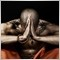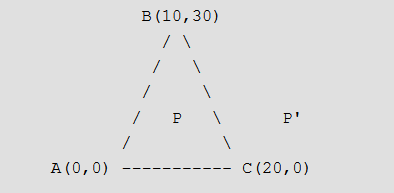# Is Bid price inside the TRIANGLE object?1568

Hi Coders,

I would like to know the position of the Bid price relative to the TRIANGLE object. Whether is it inside a TRIANGLE or not?

Do you have a simple idea for it?

Relative26114

 Relative: Bid price relative to the TRIANGLE object. Whether is it inside a TRIANGLE or not? Get the three prices from the triangle. ObjectGet - MQL4 DocumentationFind the highest and lowest.Compare them to Bid.Was that so hard?1568

WHRoeder:
 Relative: Bid price relative to the TRIANGLE object. Whether is it inside a TRIANGLE or not? Get the three prices from the triangle. ObjectGet - MQL4 DocumentationFind the highest and lowest.Compare them to Bid.Was that so hard?

I can't interpret your 1-3 points. The triangle is not a simple line. For example it can be located in the so far past too. I have to work with the price and with the time too (x, y coordinates).

Anyway, I linked a solution earlier. I just realised, somebody deleted.1568

Solution:

Given three corner points of a triangle, and one more point P. Write a function to check whether P lies within the triangle or not. For example, consider the following program, the function should return true for P(10, 15) and false for P'(30, 15)Let the coordinates of three corners be (x1, y1), (x2, y2) and (x3, y3). And coordinates of the given point P be (x, y)

1) Calculate area of the given triangle, i.e., area of the triangle ABC in the above diagram. Area A = [ x1(y2 – y3) + x2(y3 – y1) + x3(y1-y2)]/2
2) Calculate area of the triangle PAB. We can use the same formula for this. Let this area be A1.
3) Calculate area of the triangle PBC. Let this area be A2.
4) Calculate area of the triangle PAC. Let this area be A3.
5) If P lies inside the triangle, then A1 + A2 + A3 must be equal to A.Moderator
32305

WHRoeder gave the only solution possible.

Bid price only has 1 coordinate, so cannot be used as the coordinates for the corner of a triangle1568

GumRai:

Bid price only has 1 coordinate, so cannot be used as the coordinates for the corner of a triangle

The time (or number of bars) is the X coordinate.Moderator
32305

Relative:
The time (or number of bars) is the X coordinate.

Oh, I see.

Normally when you refer to Bid price it is the current price which is shown as an horizontal line across the chart.

On historical bars you would usually refer to Open, High, Low and/or Close.1568

GumRai:

Normally when you refer to Bid price it is the current price which is shown as an horizontal line across the chart.

On historical bars you would usually refer to Open, High, Low and/or Close.

X coordinate: Bars-shift (0 is the last bar, then 1, 2, 3...)

Y coordinate: Price (any price)

Acutla bid price coordinates can be for example: X:0, Y:1.12345